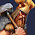## Tuesday, August 7, 2012

### Mergesort using Fork/Join Framework

The objective of this entry is to show a simple example of a Fork/Join RecursiveAction, not to delve too much into the possible optimizations to merge sort or the relative advantages of using Fork/Join Pool over the existing Java 6 based implementations like ExecutorService.

The following is a typical implementation of a Top Down Merge sort algorithm using Java:

```import java.lang.reflect.Array;

public class MergeSort {
public static <T extends Comparable<? super T>> void sort(T[] a) {
@SuppressWarnings("unchecked")
T[] helper = (T[])Array.newInstance(a.getClass() , a.length);
mergesort(a, helper, 0, a.length-1);
}

private static <T extends Comparable<? super T>> void mergesort(T[] a, T[] helper, int lo, int hi){
if (lo>=hi) return;
int mid = lo + (hi-lo)/2;
mergesort(a, helper, lo, mid);
mergesort(a, helper, mid+1, hi);
merge(a, helper, lo, mid, hi);
}

private static <T extends Comparable<? super T>> void merge(T[] a, T[] helper, int lo, int mid, int hi){
for (int i=lo;i<=hi;i++){
helper[i]=a[i];
}
int i=lo,j=mid+1;
for(int k=lo;k<=hi;k++){
if (i>mid){
a[k]=helper[j++];
}else if (j>hi){
a[k]=helper[i++];
}else if(isLess(helper[i], helper[j])){
a[k]=helper[i++];
}else{
a[k]=helper[j++];
}
}
}

private static <T extends Comparable<? super T>> boolean isLess(T a, T b) {
return a.compareTo(b) < 0;
}
}
```

To quickly describe the algorithm -
The following steps are performed recursively:

1.  The input data is divided into 2 halves
2.  Each half is sorted
3. The sorted data is then merged

Merge sort is a canonical example for an implementation using Java Fork/Join pool, and the following is a blind implementation of Merge sort using the Fork/Join framework:

The  recursive task in Merge sort can be succinctly expressed as an implementation of RecursiveAction -

``` private static class MergeSortTask<T extends Comparable<? super T>> extends RecursiveAction{
private static final long serialVersionUID = -749935388568367268L;
private final T[] a;
private final T[] helper;
private final int lo;
private final int hi;

public MergeSortTask(T[] a, T[] helper, int lo, int hi){
this.a = a;
this.helper = helper;
this.lo = lo;
this.hi = hi;
}
@Override
protected void compute() {
if (lo>=hi) return;
int mid = lo + (hi-lo)/2;
invokeAll(left, right);
merge(this.a, this.helper, this.lo, mid, this.hi);

}
private void merge(T[] a, T[] helper, int lo, int mid, int hi){
for (int i=lo;i<=hi;i++){
helper[i]=a[i];
}
int i=lo,j=mid+1;
for(int k=lo;k<=hi;k++){
if (i>mid){
a[k]=helper[j++];
}else if (j>hi){
a[k]=helper[i++];
}else if(isLess(helper[i], helper[j])){
a[k]=helper[i++];
}else{
a[k]=helper[j++];
}
}
}
private boolean isLess(T a, T b) {
return a.compareTo(b) < 0;
}
}
```

MergeSortTask above implements a compute method, which takes in a array of values, split it up into two parts, creates a MergeSortTask out of each of the parts and forks off two more tasks(hence it is called RecursiveAction!). The specific API used here to spawn of the task is invokeAll which returns only when the submitted subtasks are marked as completed. So once the left and right subtasks return the result is merged in a merge routine.

Given this the only work left is to use a ForkJoinPool to submit this task. ForkJoinPool is analogous to the ExecutorService used for distributing tasks in a threadpool, the difference to quote the ForkJoinPool's API docs:

A ForkJoinPool differs from other kinds of ExecutorService mainly by virtue of employing work-stealing: all threads in the pool attempt to find and execute subtasks created by other active tasks (eventually blocking waiting for work if none exist)

This is how the task of submitting the task to the Fork/Join Pool looks like:

``` public static <T extends Comparable<? super T>> void sort(T[] a) {
@SuppressWarnings("unchecked")
T[] helper = (T[])Array.newInstance(a.getClass() , a.length);
ForkJoinPool forkJoinPool = new ForkJoinPool(10);
1.